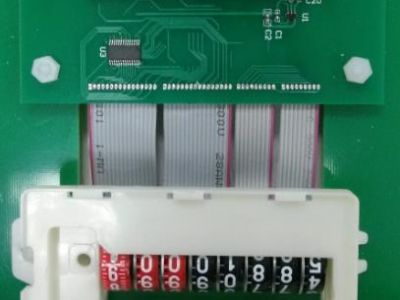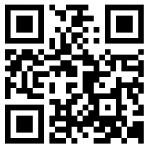Application Notes
•Watermeter DEMO Manual V2N5

For a 8-wheels meter with 10 numbers on each wheel, there are 100000000 different possibilities of number, they are 0000001, 00000002, … ,99999999. It takes huge of time to rotate and test every number possibility for every different design. So Worst case measurement is taken. Worst case happens when one wheels is being measured while other 7 wheels are aligned such that magnetic moment of the other magnet in said other 7 wheels are pointing to the same direction which gives biggest influence to the wheel being tested. If the system works in the worst case, it works in all 100000000 possibilities. There are 10 small black points near each red number 0~9 which represent the 10 numbers of the print wheel. Thus, there are 100 worst case test points in each print number, meaning 800 worst case test points in 8 print wheels totally. Below shows the 100 worst cases on print wheel #1.
•Watermeter DEMO Manual V2N4

For a 8-wheels meter with 10 numbers on each wheel, there are 100000000 different possibilities of number, they are 0000001, 00000002, … ,99999999. It takes huge of time to rotate and test every number possibility for every different design. So Worst case measurement is taken. Worst case happens when one wheels is being measured while other 7 wheels are aligned such that magnetic moment of the other magnet in said other 7 wheels are pointing to the same direction which gives biggest influence to the wheel being tested. If the system works in the worst case, it works in all 100000000 possibilities. There are 10 small black points near each red number 0~9 which represent the 10 numbers of the print wheel. Thus, there are 100 worst case test points in each print number, meaning 800 worst case test points in 8 print wheels totally. Below shows the 100 worst cases on print wheel #1.
•Watermeter DEMO Manual V2N3

For a 8-wheels meter with 10 numbers on each wheel, there are 100000000 different possibilities of number, they are 0000001, 00000002, … ,99999999. It takes huge of time to rotate and test every number possibility for every different design. So Worst case measurement is taken. Worst case happens when one wheels is being measured while other 7 wheels are aligned such that magnetic moment of the other magnet in said other 7 wheels are pointing to the same direction which gives biggest influence to the wheel being tested. If the system works in the worst case, it works in all 100000000 possibilities. There are 10 small black points near each red number 0~9 which represent the 10 numbers of the print wheel. Thus, there are 100 worst case test points in each print number, meaning 800 worst case test points in 8 print wheels totally. Below shows the 100 worst cases on print wheel #1.
•Watermeter DEMO Manual V2N2

For a 8-wheels meter with 10 numbers on each wheel, there are 100000000 different possibilities of number, they are 0000001, 00000002, … ,99999999. It takes huge of time to rotate and test every number possibility for every different design. So Worst case measurement is taken. Worst case happens when one wheels is being measured while other 7 wheels are aligned such that magnetic moment of the other magnet in said other 7 wheels are pointing to the same direction which gives biggest influence to the wheel being tested. If the system works in the worst case, it works in all 100000000 possibilities. There are 10 small black points near each red number 0~9 which represent the 10 numbers of the print wheel. Thus, there are 100 worst case test points in each print number, meaning 800 worst case test points in 8 print wheels totally. Below shows the 100 worst cases on print wheel #1.
•Watermeter DEMO Manual V2N1

For a 8-wheels meter with 10 numbers on each wheel, there are 100000000 different possibilities of number, they are 0000001, 00000002, … ,99999999. It takes huge of time to rotate and test every number possibility for every different design. So Worst case measurement is taken. Worst case happens when one wheels is being measured while other 7 wheels are aligned such that magnetic moment of the other magnet in said other 7 wheels are pointing to the same direction which gives biggest influence to the wheel being tested. If the system works in the worst case, it works in all 100000000 possibilities. There are 10 small black points near each red number 0~9 which represent the 10 numbers of the print wheel. Thus, there are 100 worst case test points in each print number, meaning 800 worst case test points in 8 print wheels totally. Below shows the 100 worst cases on print wheel #1.
• Frist
• Prev
• 1
• Next
• Last
•